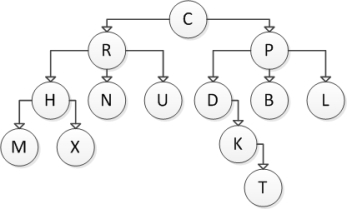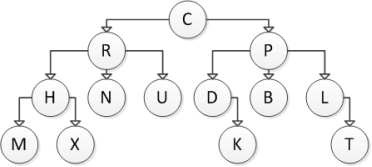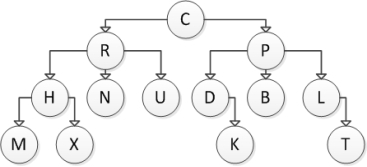# Big Java Binder Early Objects

Computing

## Quiz 17 : Tree StructuresStudy FlashcardsLooking for Programming Homework Help?

## Quiz 17 :Tree Structures

Question TypeConsider the following tree diagram:What is the size of the subtree with root R?
Free
Multiple Choice

D

Tags
Choose question tagConsider the following tree diagram:Which of the following nodes are siblings?
Free
Multiple Choice

C

Tags
Choose question tagConsider the following tree diagram:Which of the following nodes are parent nodes?
Free
Multiple Choice

D

Tags
Choose question tagConsider the following tree diagram:Which of the following nodes are child nodes?
Multiple Choice
Tags
Choose question tagConsider the following tree diagram:What is the size of this tree?
Multiple Choice
Tags
Choose question tagConsider the following tree diagram:What is the height of the subtree with root R?
Multiple Choice
Tags
Choose question tagAs implemented in the textbook, a tree class contains which of the following? I Node class II Root node III List of child nodes
Multiple Choice
Tags
Choose question tagConsider the following tree diagram:Which of the following nodes are child nodes?
Multiple Choice
Tags
Choose question tagConsider the following tree diagram:Which of the following nodes are leaf nodes?
Multiple Choice
Tags
Choose question tagGiven the Node class discussed in section 17.1 (partially shown below), select an expression to complete the isLeaf method, which is designed to return true if the node is a leaf, false otherwise. class Node { Public Object data; Public List<Node> children; ) . . Public boolean isLeaf() { Return _______________; } }
Multiple Choice
Tags
Choose question tagConsider the following tree diagram:What is the height of this tree?
Multiple Choice
Tags
Choose question tagConsider the following tree diagram:Which of the following statements is NOT correct?
Multiple Choice
Tags
Choose question tagConsider the following tree diagram:Which of the following nodes are leaf nodes?
Multiple Choice
Tags
Choose question tagConsider the following tree diagram:Which of the following statements is NOT correct?
Multiple Choice
Tags
Choose question tagConsider the following tree diagram:What is the height of the subtree with root P?
Multiple Choice
Tags
Choose question tagConsider the following tree diagram:Which of the following nodes are root nodes?
Multiple Choice
Tags
Choose question tagConsider the following tree diagram:Which of the following statements is NOT correct?
Multiple Choice
Tags
Choose question tagConsider the following tree diagram:What is the height of this tree?
Multiple Choice
Tags
Choose question tagConsider the following tree diagram:What is the size of the subtree with root P?
Multiple ChoiceConsider the following tree diagram:Which of the following nodes are interior nodes?